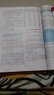# Understanding the Concept of Capacitor: Q&A

• Karan Punjabi
Basically what i am trying to say is that the voltage across the capacitor will always be the same, no matter what the voltage across the source is.f

#### Karan Punjabi

Guys, I am learning about the concept of capacitor,but I am confused in some things that if i kept the amount of charge constant between two plates of a capacitor and then increase the potential diff. this means that i decreased its capacitance right? If I am wrong then please explain the given concept that i have uploaded in the pic.

#### Attachments

•IMG_20160615_244100116.jpg
30.2 KB · Views: 397
The capacitance is a property of the circuit element in question - it's usually marked right on it. We have Q=CV, so if you increase the potential difference, the amount of charge will increase.

The capacitance is a property of the circuit element in question - it's usually marked right on it. We have Q=CV, so if you increase the potential difference, the amount of charge will increase. ...See this

No, you don't decrease the capacitance.
Why ?
If you keep the amount of charge on the capacitor constant, then you cannot change the voltage across it.
And if you change the voltage across it the charge on the plates will increase.
There is no way of circumventing that.

A little analogy:
If you had a container of water with a tube at the bottom of it, what happens when you increase the pressure in the tube ?
Water flows into the container until some point, at which the pressure due to the height is equal to the pressure in the pipe.
A capacitor works similarly. (this analogy certainly isn't perfect, especially since there are two kinds of charge and only one kind of pressure)
If you apply a voltage to a capacitor, charges will flow onto it until the voltage across it is the same as from the voltage across the source connected to it.

When you accumulate like charges in some space their potential energy increases(since they repell each other).And since voltage is just energy per charge, the (value of) voltage also increases.
So if you stuff more charge on a capacitor the voltage across it will rise, regardless of what it is connected to.
The reason why we can say ##C=\frac{Q}{V}## is that when you apply a voltage to the capacitor the amount of charge ##Q=V*C## will be on it, once it reaches equillibrium.(which it usually does insanely fast, unless you have very high resistors, impedances or capacitances in the circuit)
EDIT: I hope you understand what i am getting at.I realized that i wrote it somewhat strange and confusing.

•Karan Punjabi